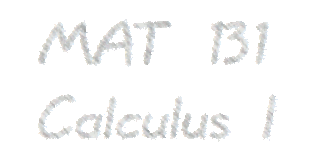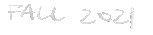# Schedule

The schedule for topics covered in lecture is as follows.
• Week 1, August 23 — August 27
Section 1.1 Review of Functions, p. 8
Section 1.2 Basic Classes of Functions, p. 36
Section 1.3 Trigonometric functions, p. 62
Appendix C Review of Pre-Calculus, p. 2017
• Week 1 Topics. Students should be able to:
Understand the concept of function, and be familiar with all the elementary functions and their graphs.
Work with piecewise functions and functions defined by tables or graphs.
Understand how functions (and their graphs) change under composition with an affine function (i.e., translations).
Work with functions, and solve algebraic equations.
Use basic modeling ideas to solve word problems.
Write and use equations of lines given two points, point and slope, etc.

• Week 2, August 30 — September 3
Section 1.4 Inverse Functions, p. 78
Section 1.5 Exponential and Logarithmic Functions, p. 96
Section 2.1 A Preview of Calculus, p. 126
Paper Homework 1 due in recitation.
• Week 2 Topics. Students should be able to:
Understand the meaning of an inverse function.
Understand the horizontal line test.
Understand how the graph of an inverse function is related to the graph of the original function.
Understand the exponential and logarithmic functions, including the exponential rules and logarithm rules.
Calculate averate rates of change for functions defined by graphs or tables.
Be able to sketch secant lines to a graph, and to use the slopes of secant lines to estimate the slope of the tangent line at a point.

• Week 3, September 6 — September 10
Section 2.2 The Limit of a Function, p. 137
Section 2.3 The Limit Laws, p. 162
Section 2.4 Continuity, p. 181
Paper Homework 2 due in recitation.
No class on Septebmer 6.
• Week 3 Topics. Students should be able to:
Understand the concept of the limit of a function for values of the input near a specified value (but not necessarily equal to that specified value).
Understand one-sided limits.
Be able to estimate such limits by inspection of the graph.
Use the "algebra of limits" to calculate limits of functions (in the case where the limit is finite only).
Understand what it means for a limit not to exist.
Calculate limits using algebra (factoring, evaluation).
Understand the statement of the squeeze theorem, and its use.
Understand the relationship between an unboundedly increasing or decreasing limit and the vertical asymptotes of the graph.
Understand the relationship between the value of a limit as the input "increases to infinity" or "decreases to negative infinity" and horizontal asymptotes of the graph.
State and understand the definition of continuity at a point and ways that a function can fail to be continuous at a point.
Define continuity on an interval.
Understand the "algebra of continuous functions".

• Week 4, September 13 — September 17
Section 2.5 The Precise Definition of a Limit, p. 196
Section 3.1 Defining the Derivative, p. 216
Paper Homework 3 due in recitation.
• Week 4 Topics. Students should be able to:
Understand the precise definition of the limit of a function.
Understand the Intermediate Value Theorem, and use it to find roots of continuous functions via repeated approximation.
Understand the meaning of the tangent to a curve at a point.
Calculate the slope of a tangent as the limit of a difference quotient.
Calculate the derivative of a given function at a point.
Estimate a derivative from a table of values of the function.
Understand velocity as a rate of change, and the difference between average and instantaneous velocity.

• Week 5, September 20 — September 24
Section 3.2 The Derivative as a Function, p. 234
Section 3.3 Differentiation Rules, p. 249
Section 3.4 Derivatives as Rates of Change, p. 268
Paper Homework 4 due in recitation.
• Week 5 Topics. Students should be able to:
Define the derivative function of a specified function.
Given the graph of a function, sketch the graph of the derivative function.
Recognize points of non-differentiability from the graph of the original function.
Calculate the second derivative function as an iterated derivative function of a specified function.
Calculate the derivatives of polynomials, sums and products, and quotients.
• Week 6, September 27 — October 1
Section 3.5 Derivatives of Trigonometric Functions, p. 280
MIDTERM 1 on Thursday, September 30. Part I in-lecture paper exam. Part II online exam beginning at 8:15pm.
No WebAssign homework due this week. No paper homework due this week.
• Week 6 Topics. Students should be able to:
Apply the mathematical notion of derivative to calculate the instantaneous rate of change of various quantities: displacement, velocity, population, revenue, etc.
Understand via a geometric argument the proof that sin(x)/x limits to 1 as x approaches 0.
Know the derivatives of the standard trigonometric functions.

• Week 7, October 4 — October 8
Section 3.6 The Chain Rule, p. 290
Section 3.7 Derivatives of Inverse Functions, p. 302
Paper Homework 5 due in recitation.
• Week 7 Topics. Students should be able to:
Understand the chain rule, and apply it in many situations.
Know the derivatives of the standard inverse trigonometric functions.
Know the derivatives of radical functions.

• Week 8, October 11 — October 15
Section 3.8 Implicit Differentiation, p. 312
Section 3.9 Derivatives of Exponential and Logarithmic Functions, p. 322
Paper Homework 6 due in recitation.
No class on October 11-12.
• Week 8 Topics. Students should be able to:
Calculate derivatives implicitly.
Use implicit differentiation to calculate slopes of implicitly defined curves and find tangent lines.
Know the derivatives of exponential and logarithmic functions.
Use the technique of logarithmic differentiation to find derivatives of large products, quotients, exponentials, etc.

• Week 9, October 18 — October 22
Section 4.1 Related Rates, p. 346
Section 4.2 Linear Approximations and Differentials, p. 359
Section 4.3 Maxima and Minima, p. 371
Paper Homework 7 due in recitation.
• Week 9 Topics. Students should be able to:
Solve related-rates problems as an application of the chain rule and implicit differentiation.
Apply linear approximations in calculations (e.g., to approximate the cube root of 9).
Estimate how errors in measurement propagate in calculations.
Locate critical points of a function; classify as to local maximum or minimum or neither.
Determine the maximum value of a function on a closed interval.

• Week 10, October 25 — October 29
Section 4.4 The Mean Value Theorem, p. 384
Section 4.5 Derivatives and the Shape of a Graph, p. 395
Section 4.6 Limits at Infinity and Asymptotes, p. 412
Paper Homework 8 due in recitation.
• Week 10 Topics. Students should be able to:
Know the statement of the Mean Value Theorem and its consequences.
Understand the relation between the second derivative and concavity of a graph.
Make accurate sketches of graphs of functions, including critical points, concavity, and inflection points.
Be able to sketch the graph of a function given the graph of the derivative function (and one point on the graph).
Use relative maxima and minima and limit information to understand functions and sketch their graphs.

• Week 11, November 1 — November 5
Section 4.7 Applied Optimization Problems, p. 444
MIDTERM 2 on Thursday, Nov 4th. Part I in-lecture paper exam. Part II online exam beginning at 8:15pm.
No WebAssign homework due this week. No paper homework due this week.
• Week 11 Topics. Students should be able to:
Translate an optimization problem into a search for the maxima/minima of a function on an interval, and translate the solution back to the original problem.

• Week 12, November 8 — November 12
Section 4.8 L'Hopital's Rule, p. 459
Section 5.1 Approximating Areas, p. 512
Section 5.2 The Definite Integral, p. 533
Paper Homework 9 due in recitation.
• Week 12 Topics. Students should be able to:
Understand when lHopitals rule can be used and how to use it (iterating if necessary).
Estimate the area under the graph of piecewise-linear function.
Estimate areas of more general (positive) functions using (finite) right-hand and left-hand sums.
Use Sigma notation for sums.
Understand the general definition of a (finite) Riemann sum.
Represent a definite integral as a limit a Riemann sum.
Extend the notion of area to "signed area" so as to include functions with negative values.

• Week 13, November 15 — November 19
Section 4.10 Antiderivatives, p. 490
Section 5.3 The Fundamental Theorem of Calculus, p. 553
Section 5.4 The Net Change Theorem, p. 570
Paper Homework 10 due in recitation.
• Week 13 Topics. Students should be able to:
Understand the definition of an antiderivative as a differentiable function whose associated derivative function is a specified function.
Recognize many antiderivative functions from derivatives studied in this course.
Understand the statement of the Fundamental Theorem of Calculus, including the formulation as the "Net Change Theorem".
Define functions in terms of definite integral, and understand the derivatives of these functions.
Combine definite integral functions with other functions, e.g., via composition, and use derivative rules and the Fundamental Theorem of Calculus to compute the derivative of the resulting functions.
Given the graph of a function, answer questions about the graph of the associated definite integral function.
Set up integrals to evaluate areas and signed areas.
Estimate distance and displacement from velocity information in a graph or table.

• Week 14, November 23 — November 26
Section 5.5 Substitution, p. 588
Section 4.9 Newton's method, p. 477
No WebAssign homework due this week. No paper homework due this week.
No class on November 24-26.
• Week 14 Topics. Students should be able to:
Compute simple integrals involving standard functions, including trigonomentric and exponential functions.
Compute integrals which result in logarithms and inverse trigonometric functions.
Compute integrals using substitution.
Use definite integrals and antiderivatives to solve simple initial-value differential equations.

• Week 15, November 29 — December 3
REVIEW
Paper Homework 11 due in recitation.

Jason Starr
4-108 Math Tower
Department of Mathematics
Stony Brook University
Stony Brook, NY 11794-3651
Phone: 631-632-8270
Fax: 631-632-7631
Jason Starr

Web design due to Scott Sutherland. Page last updated 19 August 2010 at 11:07pm.